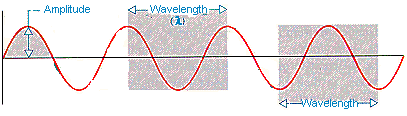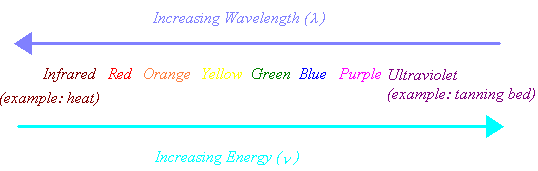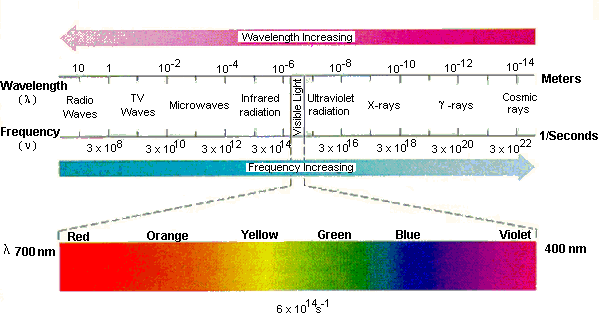Structure of the Atom

Fundamental Subatomic Particles

 Particle Symbol Charge Mass electron e- -1 0.0005486 amu proton p+ +1 1.007276 amu neutron no 0 1.008665 amu

The number of protons, neutrons, and electrons in an atom can be determined from a set of simple rules.

• The number of protons in the nucleus of the atom is equal to the atomic number (Z).
• The number of electrons in a neutral atom is equal to the number of protons.
• The mass number of the atom (M) is equal to the sum of the number of protons and neutrons in the nucleus.
• The number of neutrons is equal to the difference between the mass number of the atom (M) and the atomic number (Z).

Examples: Let's determine the number of protons, neutrons, and electrons in the following isotopes.

 12C 13C 14C 14N

The different isotopes of an element are identified by writing the mass number of the atom in the upper left corner of the symbol for the element. 12C, 13C, and 14C are isotopes of carbon (Z = 6) and therefore contain six protons. If the atoms are neutral, they also must contain six electrons. The only difference between these isotopes is the number of neutrons in the nucleus.

12C: 6 electrons, 6 protons, and 6 neutrons

13C: 6 electrons, 6 protons, and 7 neutrons

14C: 6 electrons, 6 protons, and 8 neutrons

 Practice Problem 1:Calculate the number of electrons in the Cl- and Fe3+ ions. Click here to check your answer to Practice Problem 1Much of what is known about the structure of the electrons in an atom has been obtained by studying the interaction between matter and different forms of electromagnetic radiation. Electromagnetic radiation has some of the properties of both a particle and a wave.

Particles have a definite mass and they occupy space. Waves have no mass and yet they carry energy as they travel through space. In addition to their ability to carry energy, waves have four other characteristic properties: speed, frequency, wavelength, and amplitude. The frequency (v) is the number of waves (or cycles) per unit of time. The frequency of a wave is reported in units of cycles per second (s-1) or hertz (Hz).

The idealized drawing of a wave in the figure below illustrates the definitions of amplitude and wavelength. The wavelength (l) is the smallest distance between repeating points on the wave. The amplitude of the wave is the distance between the highest (or lowest) point on the wave and the center of gravity of the wave.If we measure the frequency (v) of a wave in cycles per second and the wavelength (l) in meters, the product of these two numbers has the units of meters per second. The product of the frequency (v) times the wavelength (l) of a wave is therefore the speed (s) at which the wave travels through space.

vl = s

 Practice Problem 2:What is the speed of a wave that has a wavelength of 1 meter and a frequency of 60 cycles per second? Click here to check your answer to Practice Problem 2

 Practice Problem 3:Orchestras in the United States tune their instruments to an "A" that has a frequency of 440 cycles per second, or 440 Hz. If the speed of sound is 1116 feet per second, what is the wavelength of this note? Click here to check your answer to Practice Problem 3 Click here to see a solution to Practice Problem 3Light and Other Forms of Electromagnetic Radiation

Light is a wave with both electric and magnetic components. It is therefore a form of electromagnetic radiation.

Visible light contains the narrow band of frequencies and wavelengths in the portion of the electro-magnetic spectrum that our eyes can detect. It includes radiation with wavelengths between about 400 nm (violet) and 700 nm (red). Because it is a wave, light is bent when it enters a glass prism. When white light is focused on a prism, the light rays of different wavelengths are bent by differing amounts and the light is transformed into a spectrum of colors. Starting from the side of the spectrum where the light is bent by the smallest angle, the colors are red, orange, yellow, green, blue, and violet.

As we can see from the following diagram, the energy carried by light increases as we go from red to blue across the visible spectrum.Because the wavelength of electromagnetic radiation can be as long as 40 m or as short as 10-5 nm, the visible spectrum is only a small portion of the total range of electromagnetic radiation.The electromagnetic spectrum includes radio and TV waves, microwaves, infrared, visible light, ultraviolet, x-rays, g-rays, and cosmic rays, as shown in the figure above. These different forms of radiation all travel at the speed of light (c). They differ, however, in their frequencies and wavelengths. The product of the frequency times the wavelength of electromagnetic radiation is always equal to the speed of light.

vl = c

As a result, electromagnetic radiation that has a long wavelength has a low frequency, and radiation with a high frequency has a short wavelength.

 Practice Problem 4:Calculate the frequency of red light that has a wavelength of 700.0 nm if the speed of light is 2.998 x 108 m/s. Click here to check your answer to Practice Problem 4 Click here to see a solution to Practice Problem 4